Thermodynamics and Propulsion
Subsections

# 11.7 Performance of Propellers

In this section we will examine propeller engines. Examples of propeller-powered vehicles are shown in Figures 11.22 and 11.23.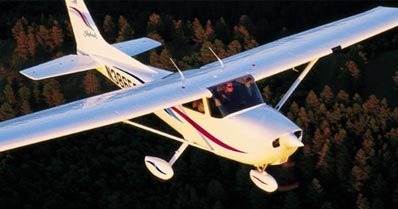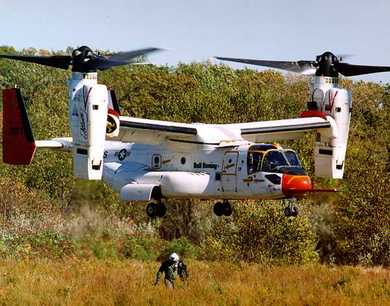## 11.7.1 Overview of propeller performance

Each propeller blade is a rotating airfoil which produces lift and drag, and because of a (complex helical) trailing vortex system has an induced upwash and an induced downwash. Figure 11.24 shows a schematic of a propeller.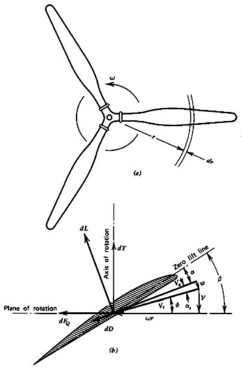The two quantities of interest are the thrust (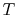)11.1 and the torque (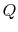). We can write expressions for these for a small radial element (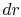) on one of the blades: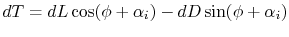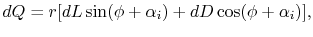where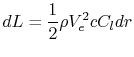and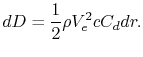It is possible to integrate the relationships as a function of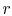with the appropriate lift and drag coefficients for the local airfoil shape, but determining the induced upwash (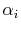) is difficult because of the complex helical nature of the trailing vortex system. In order to learn about the details of propeller design, it is necessary to do this. However, for our purposes, we can learn about the overall performance features using the integral momentum theorem, some further approximations called actuator disk theory,'' and dimensional analysis.

## 11.7.2 Application of the Integral Momentum Theorem to Propellers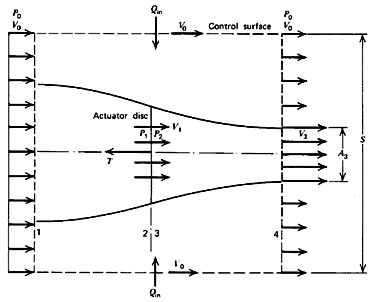The control volume shown in Figure 11.25 has been drawn far enough from the device so that the pressure is everywhere equal to a constant. This is not required, but it makes it more convenient to apply the integral momentum theorem. We will also assume that the flow outside of the propeller streamtube does not have any change in total pressure. Then since the flow is steady we apply: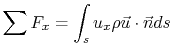Since the pressure forces everywhere are balanced, then the only force on the control volume is due to the change in momentum flux across its boundaries. Thus by inspection, we can say that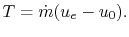We can arrive at the same result in a step-by-step manner as we did for the jet engine example previously: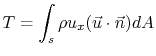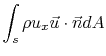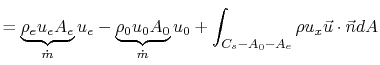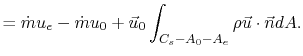Note that the last term is identically equal to zero by conservation of mass. If the mass flow in and out of the propeller streamtube are the same (as we have defined), then the net mass flux into the rest of the control volume must also be zero.

So we have: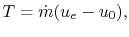as we reasoned before. The power expended is equal to the power imparted to the fluid which is the change in kinetic energy of the flow as it passes through the propeller,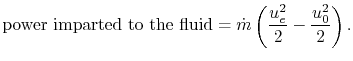The propulsive power - the rate at which useful work is done - is the thrust multiplied by the flight velocity: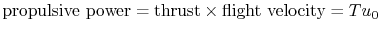The propulsive efficiency is then the ratio of these two: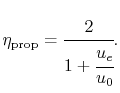This is the same expression as we arrived at before for the jet engine (as you might have expected).

## 11.7.3 Actuator Disk Theory

To understand more about the performance of propellers, and to relate this performance to simple design parameters, we will apply actuator disk theory. We model the flow through the propeller as shown in Figure 11.26 and make the following assumptions:

1. Neglect rotation imparted to the flow.
2. Assume the Mach number is low so that the flow behaves as an incompressible fluid.
3. Assume the flow outside the propeller streamtube has constant stagnation pressure (no work is imparted to it).
4. Assume that the flow is steady. Smear out the moving blades so they are one thin steady disk that has approximately the same effect on the flow as the moving blades (the actuator disk'').
5. Across the actuator disk, assume that the pressure changes discontinuously, but the velocity varies in a continuous manner.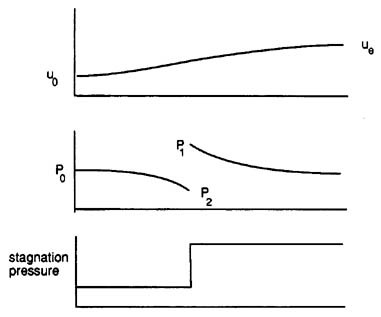We then take a control volume around the disk as shown in Figure 11.27.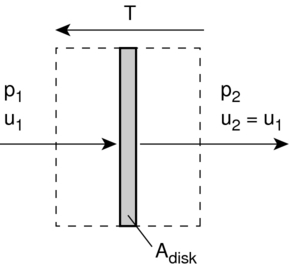The force,, on the disk is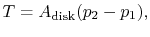so the power is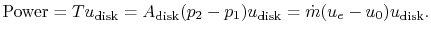We also know that the power is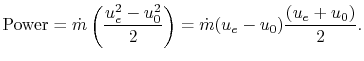Thus we see that the velocity at the disk is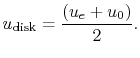Half of the axial velocity change occurs upstream of the disk and half occurs downstream of the disk.

We can now find the pressure upstream and downstream of the disk by applying the Bernoulli equation in the regions of the flow where the pressure and velocity are varying continuously: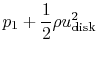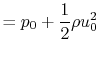and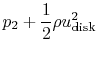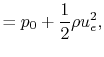from which we can determine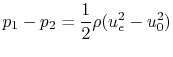We generally don't measure or control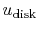directly. Therefore, it is more useful to write our expressions in terms of flight velocity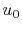, thrust,, (which must equal drag for steady level flight) and propeller disk area,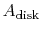.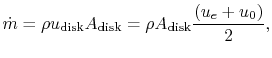so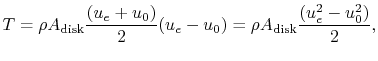from which we can obtain an expression for the exit velocity in terms of thrust and flight velocity, which are vehicle parameters: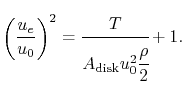The other parameters of interest become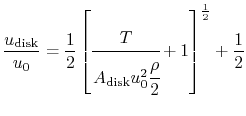and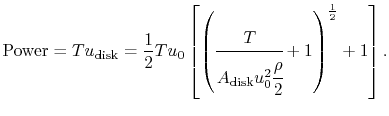This is the ideal (minimum) power required to drive the propeller. In general, the actual power required would be about 15% greater than this. Lastly, the propulsive efficiency is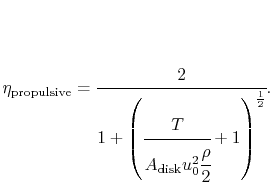There are several important trends that are apparent upon consideration of these equations. We see that the propulsive efficiency is zero when the flight velocity is zero (no useful work, just a force), and tends towards one when the flight velocity increases. In practice, the propulsive efficiency typically peaks at a level of around 0.8 for a propeller before various aerodynamic effects act to decay its performance as will be shown in the following section.

## 11.7.4 Dimensional Analysis

We will now use dimensional analysis to arrive at a few important parameters for the design and choice of a propeller. Dimensional analysis leads to a number of coefficients which are useful for presenting performance data for propellers.

 Parameter Symbol Units propeller diameter D m propeller speed n rev/s torque Q Nm thrust T N fluid densitykg/m3 fluid viscositym2/s fluid bulk elasticity modulus K N/m2 flight velocitym/s

### 11.7.4.1 Thrust Coefficient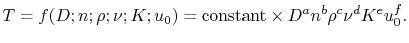Then putting this in dimensional formwhere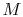is a unit of mass,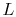is a unit of length, and hereis a unit of time. Dimensional consistency requires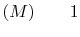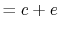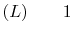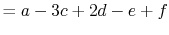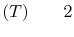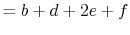So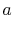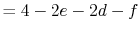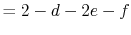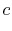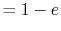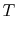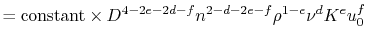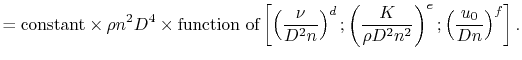We can now consider the three terms in the square brackets.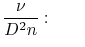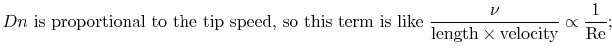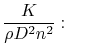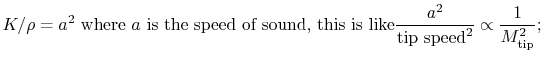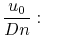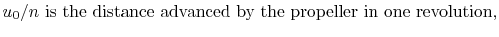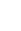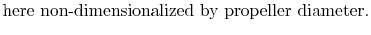This last coefficient is typically called the advance ratio and given the symbol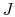. Thus we see that the thrust may be written as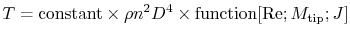which is often expressed as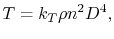where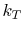is called the thrust coefficient and in general is a function of propeller design, Re,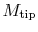and.

### 11.7.4.2 Torque Coefficient

We can follow the same steps to arrive at a relevant expression and functional dependence for the torque or apply physical reasoning. Since torque is a force multiplied by a length, it follows that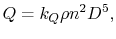where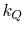is called the torque coefficient and in general is a function of propeller design, Re,, and.

### 11.7.4.3 Efficiency

The power supplied to the propeller is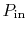where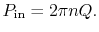The useful power output is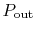where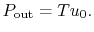Therefore the efficiency is given by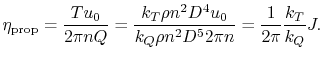### 11.7.4.4 Power Coefficient

The power required to drive the propeller is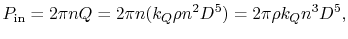which is often written using a power coefficient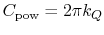,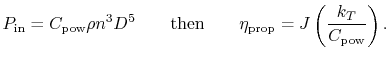### 11.7.4.5 Typical propeller performance

Typical propeller performance curves are shown in Figures 11.28, 11.29, and 11.30.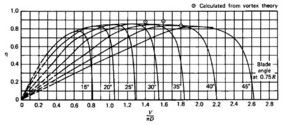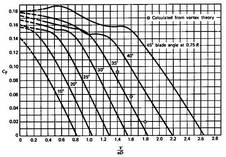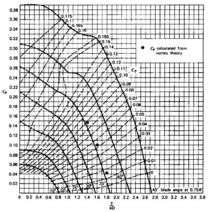UnifiedTP